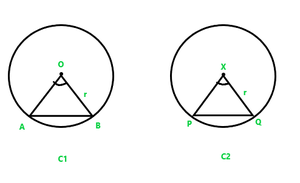Open in App
Not now

## Related Articles

• NCERT Solutions for Class 9 Maths

# Class 9 NCERT Solutions – Chapter 10 Circles – Exercise 10.2

• Last Updated : 20 Dec, 2021

### Question 1. Recall that two circles are congruent if they have the same radii. Prove that equal chords of congruent circles subtend equal angles at their centres.

Solution:Given:

Two Congruent Circles C1 and C2

AB is the chord of C1

and PQ is the chord of C2

AB = PQ

To Prove: Angle subtended by the Chords AB and PQ are equal i.e. ∠AOB = ∠PXQ

Proof:

In △AOB & △PXQ

AO = PX                    (Radius of congruent circles are equal)

BO = QX                      (Radius of congruent circles are equal)

AB = PQ                    (Given)

△AOB ⩭ △PXQ       (SSS congruence rule)

Therefore, ∠AOB = ∠PXQ         (CPCT)

### Question 2. Prove that if chords of congruent circles subtend equal angles at their centres, then the chords are equal.

Solution:Given:

Two Congruent circles C1 and C2

AB is the chord of C1 and PQ is chord of C2

& ∠AOB = ∠PXQ

To prove :

In △AOB and  △PXQ ,

AO = PX                         (Radius of congruent circles are equal)

∠AOB = ∠PXQ              (Given)

BO = QX                          (Radius of congruent circles are equal)

△AOB ⩭ △PXQ          (SAS congruence rule)

Therefore, AB = PQ       (CPCT)

My Personal Notes arrow_drop_up